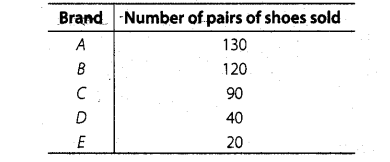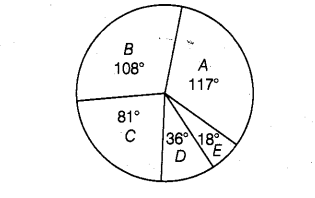# Shoes of the following brands are sold

Question:

Shoes of the following brands are sold in November 2007 at a shoe store. Construct a pie chart for the given data.Solution:

Total number of pairs of shoes sold $=(130+120+90+40+20)=400$ $\therefore$ Central angle of pie chart representing the brand

(i) $A=\frac{130}{400} \times 360^{\circ}=117^{\circ}$

(ii) $B=\frac{120}{400} \times 360^{\circ}=108^{\circ}$

(iii) $C=\frac{90}{40} \times 360^{\circ}=81^{\circ}$

(iv) $D=\frac{40}{400} \times 360^{\circ}=36^{\circ}$

(v) $E=\frac{20}{400} \times 360^{\circ}=18^{\circ}$